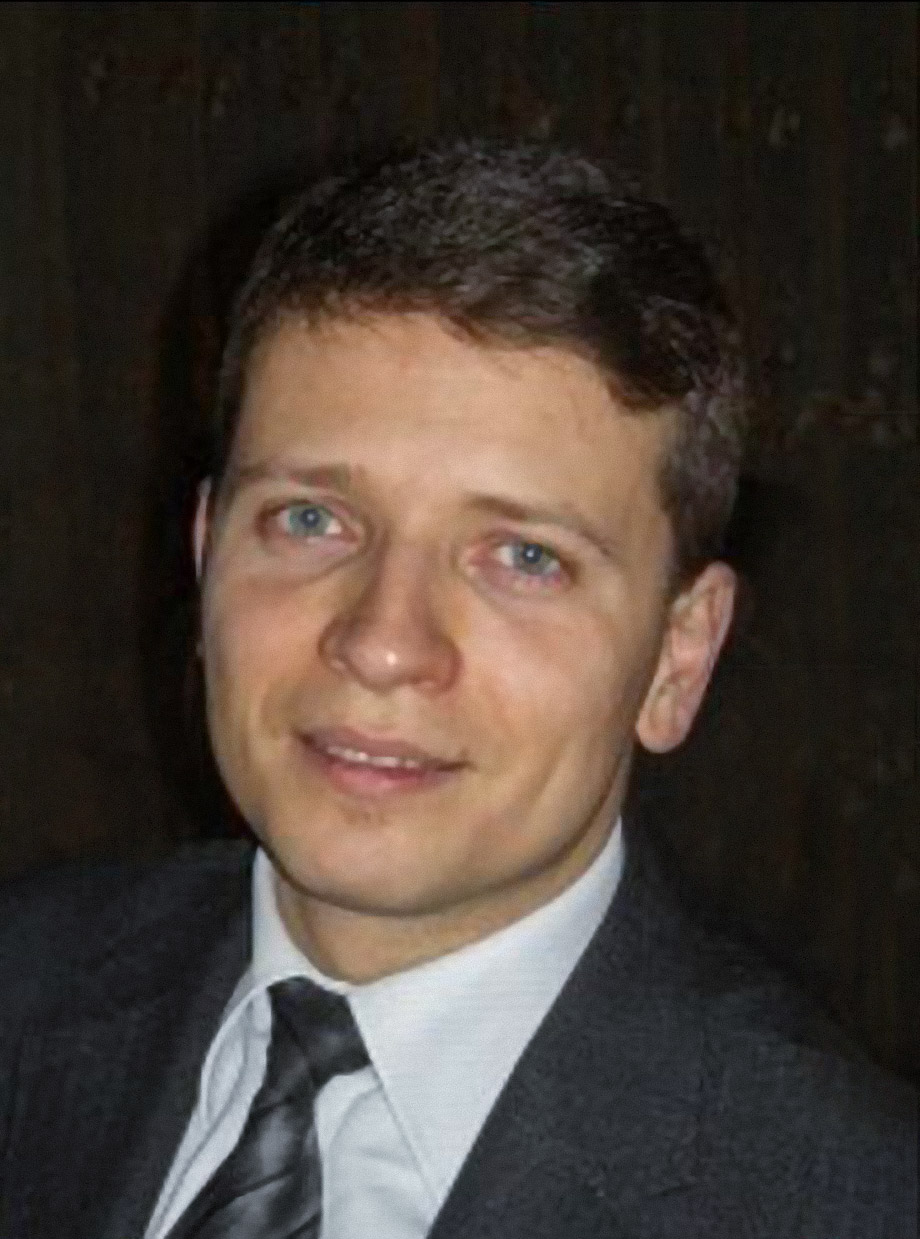﻿ Laboratory site
En / Ru## Solodov Aleksei Petrovich

→ Deputy Head of the Laboratory

→ Assistant Professor of the Department of Mathematical Analysis, Faculty of Mechanics and Mathematics, Lomonosov Moscow State University

→ Deputy Head of the Department of Methodology of Teaching Elementary Mathematics at the Department of Mathematical Analysis, Lomonosov Moscow State University

E-mail:

Keywords: generalized integrals, Saks–Henstock lemma, sine series with monotone coefficients, orthonormal system, Rademacher–Menchov theorem, Weyl multiplier, domains of univalence of holomorphic maps, fixed points, angular derivative.

Education:

→ 1991-1996 – Faculty of Mechanics and Mathematics, Lomonosov Moscow State University;

→ 1996-1999 – graduate school of the Department of Theory of Functions and Functional Analysis, Faculty of Mechanics and Mathematics, Lomonosov Moscow State University, advisor Prof. V.A. Skvortsov;

→ 1999 – PhD Thesis "Generalized Integration of Banach-Valued Functions" (Faculty of Mechanics and Mathematics, Lomonosov Moscow State University).

→ 2021 – Dr. Sci. Thesis "Extremal problems in the theory of orthogonal series and complex analysis" (Steklov Institute).

### Research Interests:

→ Generalized integrals, trigonometric series with monotone coefficients, orthogonal series, geometric theory of functions of a complex variable.

### Educational and methodical activity:

→ Specialist in the teaching of school mathematics and mathematical analysis at primary courses of universities.

→ Chairman of jury organizing committees of school olympiads passing in Lomonosov Moscow State University.

→ Head of a methodical seminar of the Department of Mathematical Analysis.

### Publications (recent):

2023

→ M. I. Dyachenko, A. P. Solodov, "Asymptotics of Sums of Sine Series with Fractional Monotonicity Coefficients", Analysis Mathematica, 49:1 (2023), 67–77, DOI.

→ O. S. Kudryavtseva, A. P. Solodov, "Estimate of the Second Coefficient of Holomorphic Mappings of a Disk into Itself with Two Fixed Points", Math. Notes, 113:5 (2023), 694–699, DOI.

→ T. P. Lukashenko, V. A. Skvortsov, A. P. Solodov, "Generalized integrals", Lenand, Moscow, 2023, 320 pp.

2022

→ V. V. Goryainov, O. S. Kudryavtseva, A. P. Solodov, "Iterations of holomorphic mapc, fixed points, and univalence domains", Uspekhi Mat. Nauk, 77:6(468) (2022), 3–68, DOI.

→ A. Yu. Popov, A. P. Solodov, "Optimal Two-Sided Estimates on the Interval $$[\frac{\pi}{2},\pi]$$ of the Sum of the Sine Series with Convex Coefficient Sequence", Math. Notes, 112:2 (2022), 328–331, DOI.

→ O. S. Kudryavtseva, A. P. Solodov, "Generalization of the Landau and Becker–Pommerenke inequalities", Dokl. Math. 106 (2022), 251–253, DOI.

→ O. S. Kudryavtseva, A. P. Solodov, "An inverse function theorem on the class of holomorphic self-maps of a disc with two fixed points", Uspekhi Mat. Nauk, 77:1(463) (463) (2022), 187–188, DOI.

→ Al. R. Valiullin, Ar. R. Valiullin, A. P. Solodov, "Sharp sufficient condition for the convergence of greedy expansions with errors in coefficient computation", Demonstratio Mathematica, 55:1 (2022), 254–264, DOI.

2021

→ A. P. Solodov, "The exact domain of univalence on the class of holomorphic maps of a disc into itself with an interior and a boundary fixed points", Izv. Math., 85:5 (2021), 1008–1035, DOI.

→ A. P. Solodov, "Sharp two-sided estimate for the sum of a sine series with convex slowly varying sequence of coefficients", Analysis Mathematica, 46:3 (2020), 579–603, DOI.

→ O. Kudryavtseva, A. Solodov, "On the Boundary Dieudonné–Pick Lemma", Mathematics, 9:10 (2021), 1108, DOI.

→ A. P. Solodov, "On Orthogonal Systems with Extremely Large $$L_2$$-Norm of the Maximal Operator", Math. Notes, 109:3 (2021), 459–472, DOI.

2020

→ A. P. Solodov, "Strengthening of Landau's Theorem for Holomorphic Self-Mappings of a Disk with Fixed Points", Math. Notes, 108:4 (2020), 626–628, DOI.

→ A. P. Solodov, "Exact Constants in Telyakovskii's Two-Sided Estimate of the Sum of a Sine Series with Convex Sequence of Coefficients", Math. Notes, 107:6 (2020), 958–971, DOI.

→ O. S. Kudryavtseva, A. P. Solodov, "Asymptotically sharp two-sided estimate for domains of univalence of holomorphic self-maps of a disc with an invariant diameter", Mat. Sb., 211:11 (2020), 96–117; English translation in Sb. Math., 211:11 (2020), 1592—1611, DOI.

2019

→ O. S. Kudryavtseva, A. P. Solodov, "Two-sided estimate of univalence domains of holomorphic mappings of the disc into itself with an invariant diameter", Russian Math., 63:7 (2019), 80–83, DOI.

→ O. S. Kudryavtseva, A. P. Solodov, "Two-sided estimates for domains of univalence for classes of holomorphic self-maps of a disc with two fixed points", Sb. Math., 210:7 (2019), 1019–1042, DOI.

2018

→ A. Yu. Popov, A. P. Solodov, "Estimates with Sharp Constants of the Sums of Sine Series with Monotone Coefficients of Certain Classes in Terms of the Salem Majorant", Mat. Zametki, 104:5 (2018), 725–736; English translation in Math. Notes, 104:5 (2018), 702–711, DOI.

→ A. P. Solodov, "Asymptotic behavior of the sum of sines series in the zero neighborhood", Bulletin of the L.N. Gumilyov Eurasian National University. Mathematics. Computer science. Mechanics Series, 125:4 (2018), 108–112.

2017

→ A. Yu. Popov, A. P. Solodov, "The negative parts of the sums of sine series with quasimonotonic coefficients", Mat. Sb., 208:6 (2017), 146–169; English translation in Sb. Math., 208:6 (2017), 878–901, DOI.